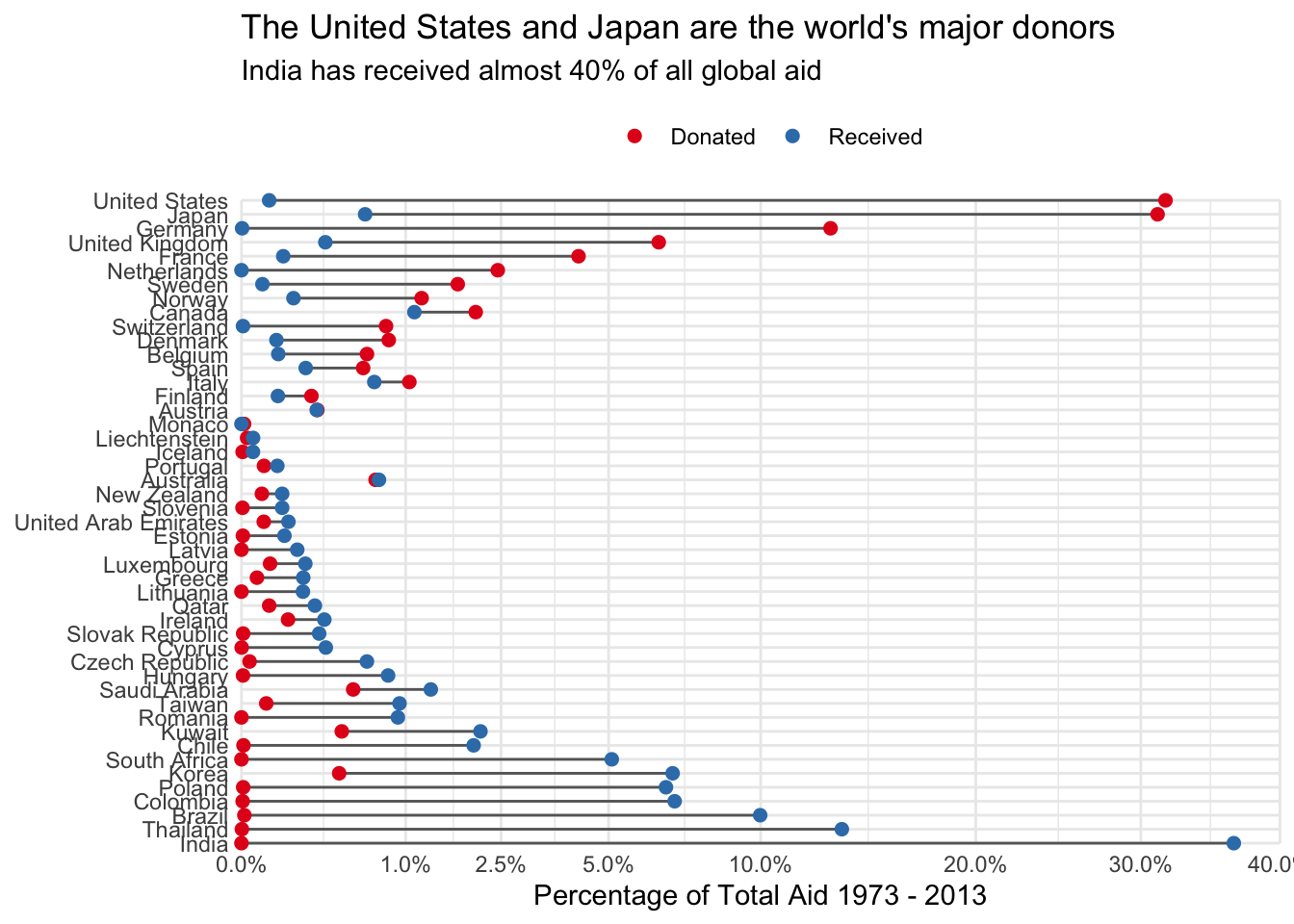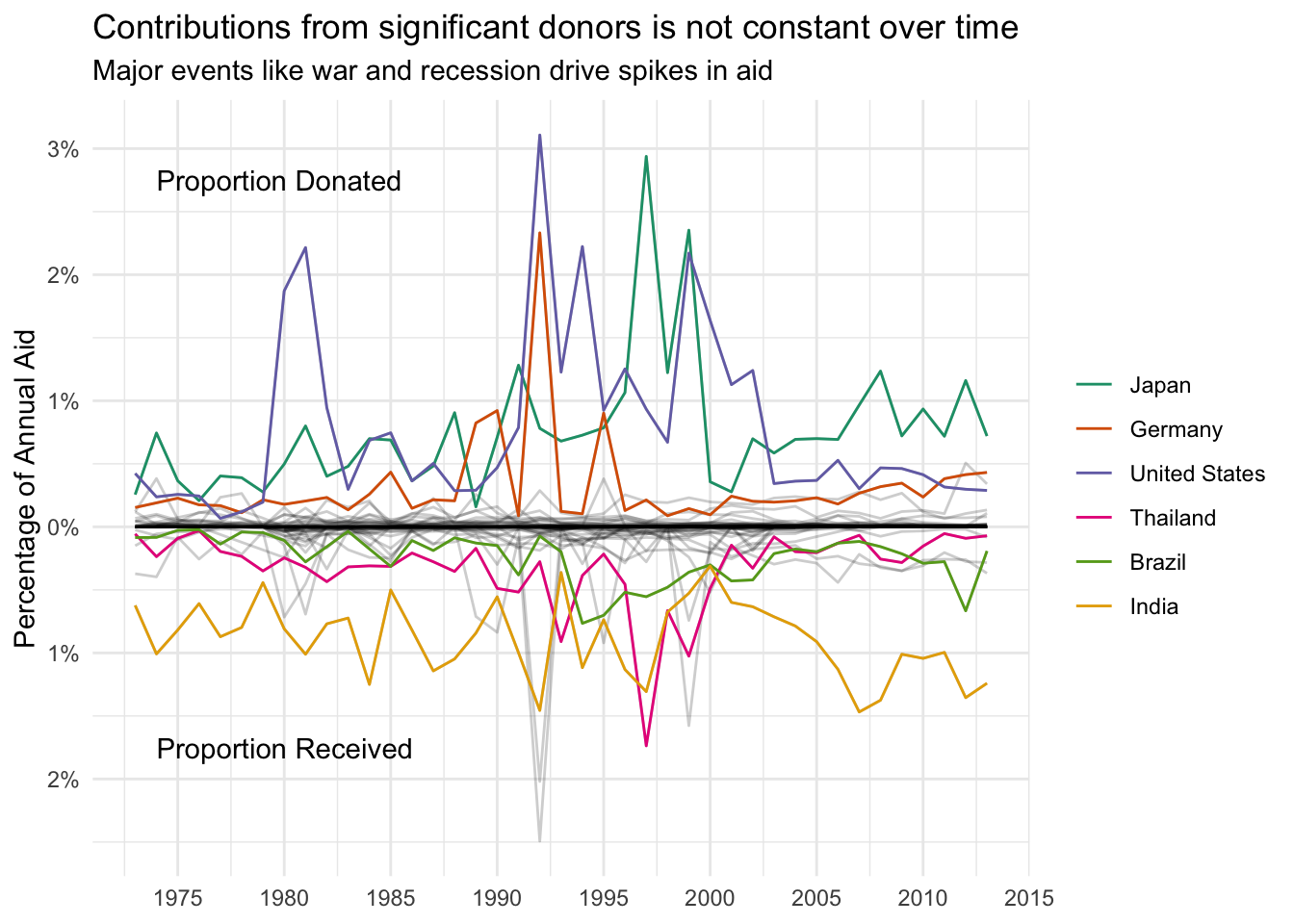# #SWDChallenge March 2019

This month I decided to take part in the Storytelling With Data monthly challenge for the first time! The dataset we were given to explore contains global aid exchanges between 47 countries across the world across the years 1973-2013. The goal is to create visualizations that answers the broad question: Who Donates?, as well as some bonus questions about distribution of donations geographically, temporally and by purpose of donation. Here’s my initial attempt! Along with some code. (The only package I use here is `tidyverse`).

### The Data

The data provided is nice and clean, so all we are left to do is read it in using `read_csv()`. I changed some variable names to make it nicer to work with, and noticed that there are a few negative quantities of money in the data, which I drop since they are impossible. Here’s a glimpse of what the data looks like:

id year donor recipient amount purpose_code purpose_desc
2414478 1977 Saudi Arabia India 348718518 23030 Power generation/renewable sources
2414509 1977 Saudi Arabia Brazil 191647004 23040 Electrical transmission/ distribution
2414635 1983 Saudi Arabia India 79371799 21030 Rail transport
2414665 1984 Saudi Arabia Taiwan 212202942 21030 Rail transport
2414667 1984 Saudi Arabia Korea 134511154 21040 Water transport
2414684 1985 Saudi Arabia India 128074768 23000 Energy generation and supply, combinations of activities

### Who Donates?

One of the challenges in answering this question is how to summarize across time. I chose to look at the proportion of the total money contributed to global aid that each country contributed and received.

``````donated <-
data %>%
group_by(donor) %>%
summarise(donated = sum(amount)) %>%
mutate(prop_donated = donated / sum(donated)) %>%
select(country = donor, prop_donated)``````
``## `summarise()` ungrouping output (override with `.groups` argument)``
``````received <-
data %>%
group_by(recipient) %>%
``## `summarise()` ungrouping output (override with `.groups` argument)``
``````aid <-
donated %>%
mutate_at(vars(-country), ~if_else(is.na(.), 0, .)) %>%
gather(-country, key = direction, value = proportion_of_aid) %>%
mutate(direction = str_extract(direction, "[^_]+\$"))

country_order <-
aid %>%
mutate(diff = donated - received) %>%
arrange(diff) %>%
pull(country)

aid <-
aid %>%
mutate(country = factor(country, levels = country_order, ordered = TRUE))

segments <-
aid %>%

aid %>%
ggplot(aes(y = country)) +
geom_segment(
aes(yend = country, x = donated, xend = received),
color = "grey40",
data = segments
) +
geom_point(aes(x = proportion_of_aid, color = direction), size = 2) +
scale_y_discrete(expand = expand_scale(0)) +
scale_x_sqrt(
labels = scales::percent,
expand = expand_scale(0),
limits = c(0,0.4),
breaks = c(0, 0.01, 0.025, 0.05, 0.1, 0.2, 0.3, 0.4)
) +
scale_color_brewer(type = "qual", palette = "Set1", labels = str_to_title) +
theme_minimal() +
theme(legend.position = "top") +
coord_cartesian(clip = "off") +
labs(
y = NULL,
x = glue::glue("Percentage of Total Aid {min(pull(data, year))} - {max(pull(data, year))}"),
color = NULL,
title = "The United States and Japan are the world's major donors",
subtitle = "India has received almost 40% of all global aid"
)``````
``````## Warning: `expand_scale()` is deprecated; use `expansion()` instead.

## Warning: `expand_scale()` is deprecated; use `expansion()` instead.``````Excuse the squished y-axis. I played around with it for a while and eventually gave up. Any hints are very welcome!

### Has the Amount Donated Changed Over Time?

In keeping with the same metric, proportion of aid contributed and received, we can also look at the trends over time. I’ve highlighted the top three donors and recipients in the figure. Interestingly, it seems that receiving tends to be steadier over time, while donations see more anomalous spikes

``````donated <-
data %>%
group_by(donor, year) %>%
summarise(donated = sum(amount)) %>%
ungroup() %>%
mutate(prop_donated = donated / sum(donated)) %>%
select(country = donor, year, prop_donated)``````
``## `summarise()` regrouping output by 'donor' (override with `.groups` argument)``
``````received <-
data %>%
group_by(recipient, year) %>%
ungroup() %>%
``## `summarise()` regrouping output by 'recipient' (override with `.groups` argument)``
``````timeseries <-
donated %>%
full_join(received, by = c("country", "year")) %>%
mutate_at(vars(prop_donated, prop_received), ~if_else(is.na(.), 0, .)) %>%
gather(prop_donated, prop_received, key = direction, value = proportion) %>%
mutate(
proportion = if_else(direction == "prop_donated", proportion, -proportion)
)

top_3 <-
aid %>%
filter(direction == "donated") %>%
top_n(3, proportion_of_aid) %>%
pull(country)

bottom_3 <-
aid %>%
top_n(3, proportion_of_aid) %>%
pull(country)

timeseries_main <-
timeseries %>%
filter(country %in% top_3 & direction == "prop_donated" |
country %in% bottom_3 & direction == "prop_received")

country_order <-
timeseries_main %>%
filter(year == max(pull(data, year))) %>%
arrange(desc(proportion)) %>%
pull(country)

timeseries_main <-
timeseries_main %>%
mutate(country = factor(country, levels = country_order, ordered = TRUE))

labeller <-
function(y) {
y = if_else(y < 0, -y, y)
scales::percent(y)
}

timeseries %>%
unite(group, country, direction, remove = FALSE) %>%
ggplot(aes(year, proportion)) +
geom_line(aes(group = group), alpha = 0.2) +
geom_line(aes(color = country), data = timeseries_main) +
scale_x_continuous(breaks = seq(1970, 2015, by = 5)) +
scale_y_continuous(labels = labeller) +
scale_color_brewer(type = "qual", palette = "Dark2") +
theme_minimal() +
labs(
x = NULL,
color = NULL,
y = glue::glue("Percentage of Annual Aid"),
title = "Contributions from significant donors is not constant over time",
subtitle = "Major events like war and recession drive spikes in aid"
) +
annotate(
geom = "text",
x = 1974,
y = 0.0275,
label = "Proportion Donated",
hjust = 0
) +
annotate(
geom = "text",
x = 1974,
y = -0.0175,